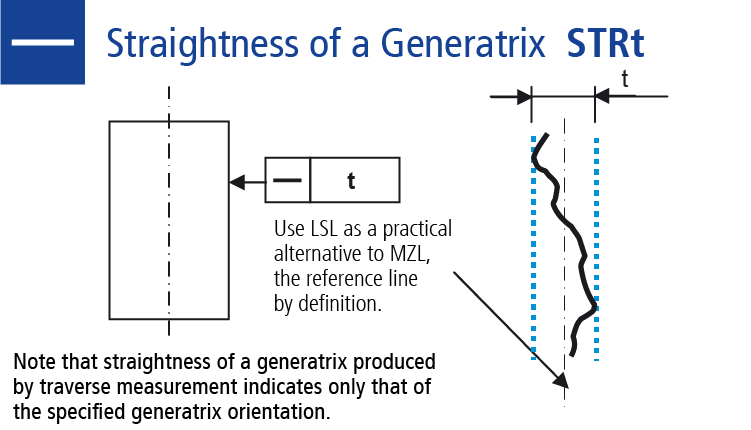•# Measure the Straightness (STRt)

The straightness of the shape tolerance measures the deviation of a measured straight line from the geometrically ideal straight line. The toleranced elements are the straights or axis.

Straightness tolerance of an axis:
The tolerance zone is limited by a cylinder at diameter t.

Straightness tolerance of an edge:
The tolerance zone is limited by two parallel, straight planes at distance t.

Straightness tolerance of a mantle line:
Each mantel line of the tolerated cylindrical surfaces must lie between two parallel straights lines at distance t.